## Section58.5Particle Production and Detection

### Subsection58.5.1Particle Production

Initially particle physics was studied using naturally occurring particles such as electrons, protons, and neutrons in atoms, particles in cosmic rays, and particles generated by the interaction of cosmic rays with the atmosphere. The testing of standard model of particle physics requires production of particles of very high rest mass. They are produced in specially designed accelerators. We will first discuss the energy required to produce a particle of particular rest mass.

#### Subsubsection58.5.1.1Energy Required to Produce an Elementary Particle

Elementary particles beyond the mass of neutron are usually unstable and are normally not found in nature. To study them or to even check for their existence we need to synthesize in the laboratory. The most fruitful method for synthesis has been to accelerate a charged particles such as an electron or proton and smash them on a target. If the energy is high enough the destruction of particles sometimes leads to the production of the desired particles.

Colliding two particles moving in opposite directions

Suppose we smash two particles of equal mass $m\text{,}$ say two protons, at each other with equal and opposite momentum $p$ for simplicity. We wish to have enough energy to produce a particle of mass $M$ at rest after the event. Since we deal with highly relativistic particles, we will work using relativistic expressions. The conservation of energy will give us

\begin{equation} 2(K + m c^2)= Mc^2,\tag{58.5.1} \end{equation}

where

\begin{equation*} K = \sqrt{p^2c^2 + m^2 c^4} - m c^2. \end{equation*}

Therefore, the kinetic energy of each of the colliding particles must be at least the following value.

\begin{equation*} K = \left(\frac{M}{2} - m\right)\: c^2 \end{equation*}

If the kinetic energy is greater than this value, the particle $M$ will be produced with non-zero kinetic energy.

A moving particle incident on fixed target

Suppose a particle of mass $m_1$ and momentum $p_1$ is incident on a particle of mass $m_2$ at rest and a particle of mass $M$ is produced with momentum $p$ in the lab frame. We define threshold energy $K_{th}$ as the kinetic energy in the lab frame that corresponds to the particle M at rest in the Center of Momentum (CM) frame. We will not carry out the proof but one can show that the threshold energy $K_{th}$ to produce the particle $M$ is given by

\begin{equation} K_{th} = \frac{M^2c^2 - (m_1 + m_2)^2c^2}{2m_2}. \label{eq-particle-production-thresh}\tag{58.5.2} \end{equation}

A proton beam strikes a Lithium nucleus at rest. What will be the threshold energy of the proton to produce $Z^{0}$ boson. Data: Rest mass of proton 938.3 MeV/c$^2\text{,}$ Lithium 26 GeV/c$^2\text{,}$ $Z^{0}$ 91.2 GeV/c$^2\text{.}$

Hint

Use Eq. (58.5.2).

$146\:\textrm{GeV}\text{.}$

Solution

Using Eq. (58.5.2) we find

\begin{equation*} K_{th} = \frac{91.2^2 - 26.9383^2}{2\times 26}\:\textrm{GeV} = 146\:\textrm{GeV} . \end{equation*}

### Subsection58.5.2Particle Detection

Progress in particle production and detection methods have played crucial role in our understanding of the fundamental physics. The development of detector technologies mirrors well the advancement of fundamental physics as shown in Table 58.5.2, adapted from: W. Riegler, Particle Detectors, CERN.

Particle detectors can be broadly classified into counters and track detectors. In particle counters we are mostly interested in the intensity of the particles.

### Subsection58.5.3Counters

#### Subsubsection58.5.3.1Ion chamber

An ion chamber detector is based on the fact that if a highly energetic particle passes through a gas, the gas is ionized with electron-ion pair production if the particle is capable of electromagnetic interaction.

\begin{equation*} M + \gamma \longrightarrow\ M^{+} + e^{-} + \gamma^{*},\ \ \ M + p \longrightarrow\ M^{+} + e^{-} + p^{*},\ \ \ \textrm{etc,} \end{equation*}

where $\gamma$ and $p$ are a photon and a proton of sufficiently high energy to ionize the molecule $M\text{.}$ The $\gamma^{*}$ and $p^{*}$ in the product are photon and proton with less energy than the corresponding photon and proton on the reactant side. In an ionization chamber two plates in a chamber are maintained at different voltages as shown in Figure 58.5.3.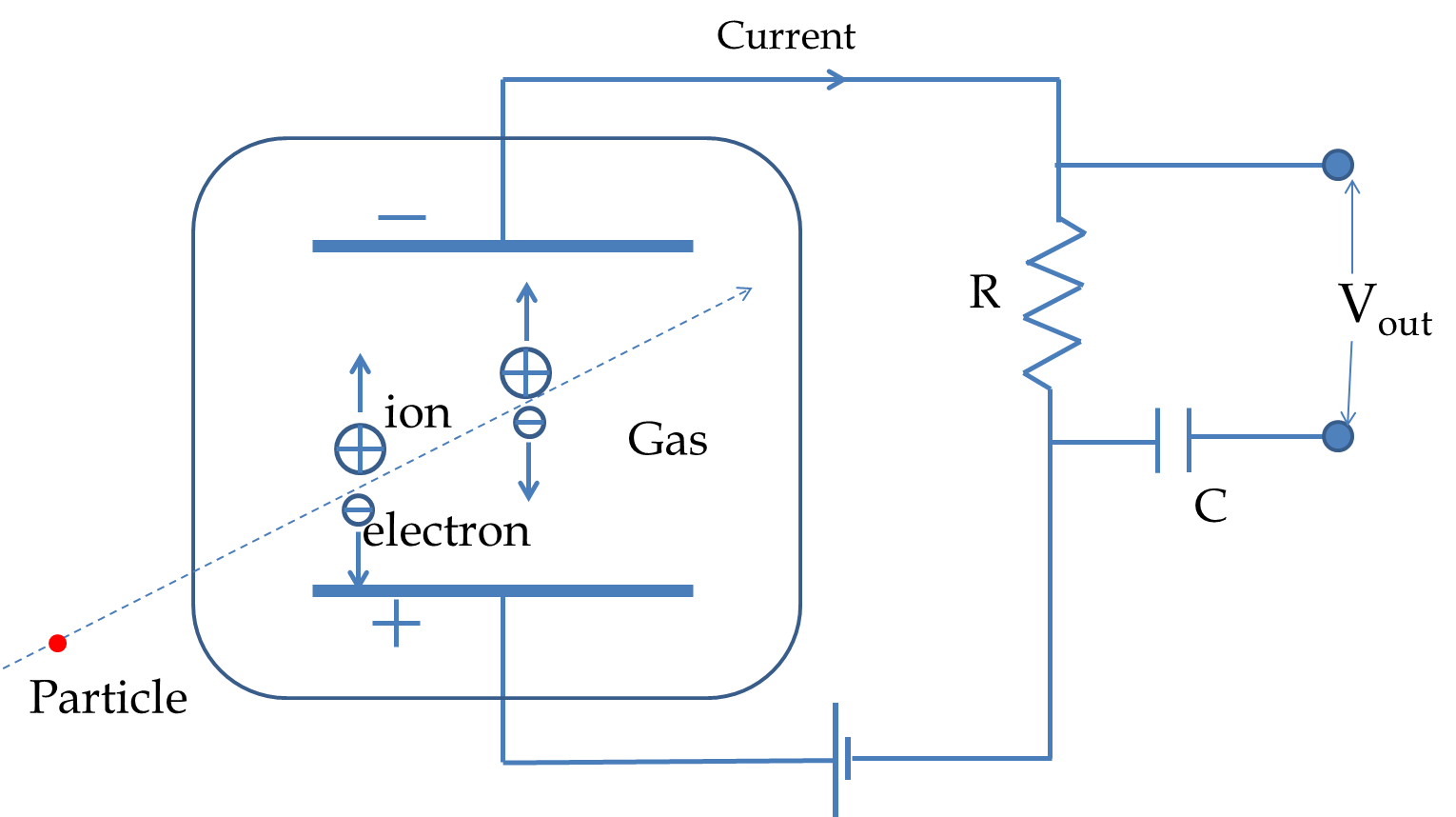Figure 58.5.3. A particle entering in the ion chamber generates ion-electron pair. The number of pairs generated is proportional to the kinetic energy of the particle, and hence the current in the circuit is proportional to the kinetic energy of the particle. Usually, the output voltage is amplified by an amplifier circuit not shown here.

When an energetic particle enters the chamber and is stopped by the molecules, the number of ion-electron pair generated is proportional to the kinetic energy of the particle. The electrons are attracted towards the positive plate and the positive ions towards the negative plate creating a current in the circuit that is proportional to the kinetic energy of the particle.

\begin{equation*} \textrm{Current}\ \propto\ \textrm{Kinetic energy of particle if particle is stopped in the chamber.} \end{equation*}

If the particle is too energetic, we can only detect the presence or absence of the particle since the emerging particle will carry away some kinetic energy. When the particle is stopped in the chamber, we can obtain both the presence of a particle and its energy. The ion chamber is then called a proportional counter.

The Geiger-Mueller (GM) tube of the familiar Geiger counter used for detection of radiation is an ionization chamber. In a GM tube the ionization chamber is a cylindrical tube, which acts as the negative plate with a central wire which acts as the positive plate. The ionizing particles enter the Geiger tube through a thin wall. The ionized gas molecules are attracted towards the wall and the electrons to the central positive wire, which is maintained 1000 V or so above the wall. The accelerating electrons knock out electrons from other molecules which amplifies the signal in an avalanche process illustrated in Figure 58.5.4. The current from the GM tube is not directly proportional the the energy of the ionizing particle. Therefore, although a Geiger counter can detect particles quite efficiently, it is not useful for obtaining the energy of the particle.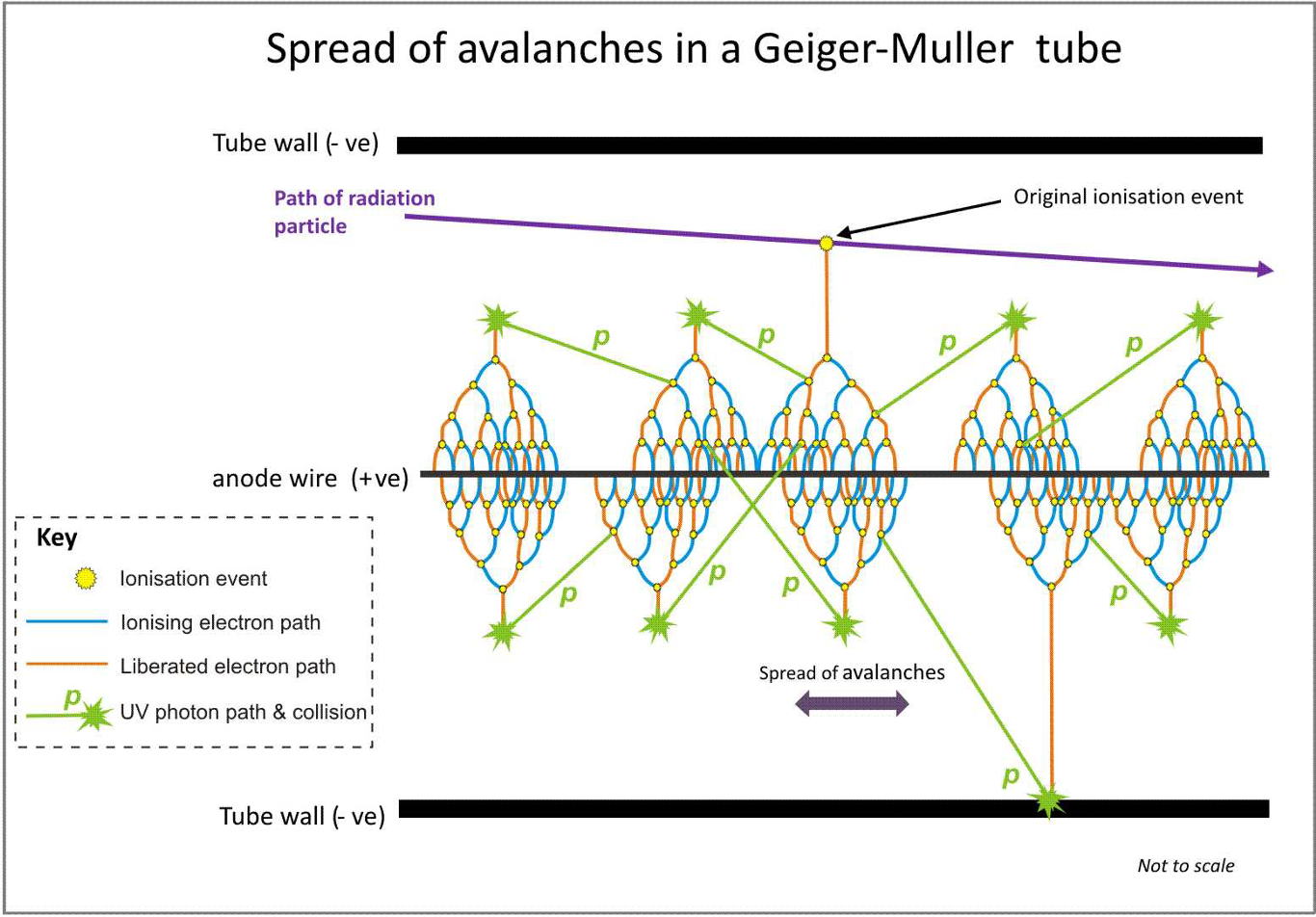Figure 58.5.4. The schematics of a Geiger-Mueller tube. The electrons released from the ionization of molecules of the gas in a rush towards the central wire ionize more molecules in an avalanche process. Credits: Wikicommons

The smoke detector is an example of an ionization chamber which has an open chamber. The chamber usually contains the radioactive isotope $^{241}$Am, which is an alpha emitter and produces a steady current. When smoke enters the chamber, the smoke molecules prevent the flow of ions to the negative plate which gets picked up by the detector.

#### Subsubsection58.5.3.2Scintillation counter

Scintillation counters are more sensitive equipment than ionization chambers. In a scintillation counter a high energy particle enters in a medium, called a scintillator, whose molecules are excited and emit light. i.e. glow. The most common material for scintillation in particle physics is the NaI crystal. The light from the scintillator is then reflected in a photomultiplier tube, which is a highly sensitive equipment. The first stage of the photomultiplier is a photocathode which releases electrons when light is incident on it. The electrons are then accelerated and strike plates that are at successively higher voltages. At each strike, more electrons are released, and hence considerable amplification can be achieved from a few at the photocathode.

If the particle stops in the scintillating material, then the current detected by the photomultiplier tube is proportional to the kinetic energy of the particle. In this way, we can both detect a particle and measure its energy as well.

### Subsection58.5.4Track Detectors

We have already seen some results of track detectors in Figure 58.1.1 and Figure 58.1.2. Track detectors record the path of particles. If a particle is electrically charged the path can be varied by electric and magnetic field. The radius of curvature $R$ of the track of a particle of charge $q$ in a magnetic field gives information about the momentum $p$ of the particle as the following $F = m a$ of a charged particle in a magnetic field perpendicular to the velocity of the particle.

\begin{equation*} qvB = mv^2/R\ \ \Longrightarrow\ \ \ p = q B R. \ \ \ (B\ \textrm{perpendicular to track}.) \end{equation*}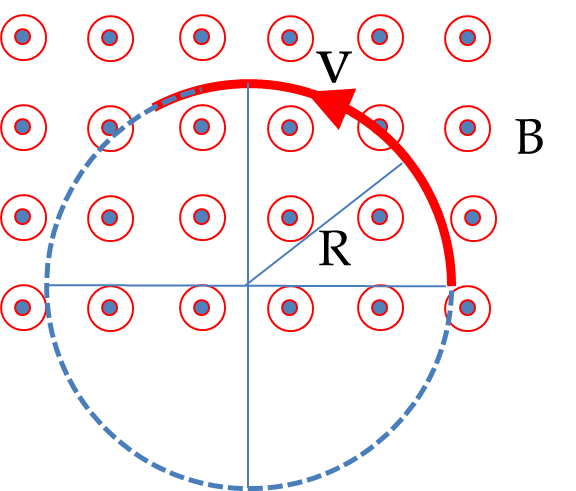Figure 58.5.6. The radius of curvature of a track of a charged particle in a magnetic field is used to determine the momentum of the particle by $p = q B R\text{.}$

Another important technique is the use of Cerenkov radiation to determine the velocity of an electrically charged particle. If a particle has speed in a dielectric medium greater than the speed of light in that medium, then light is emitted in the shape of a cone as illustrated in Figure 58.5.7. Cerenkov radiation can be seen in the blue glow inside a reactor as shown in Figure 58.5.8. The angle of the cone $\theta$ gives information about the speed of the particle as obtained from the geometry in Figure 58.5.7.

\begin{equation*} \cos\theta = \frac{(c/n) t}{v t} = \frac{c}{n v},\ \ \Longrightarrow\ \ \ v = \frac{c}{n\cos\theta}. \end{equation*}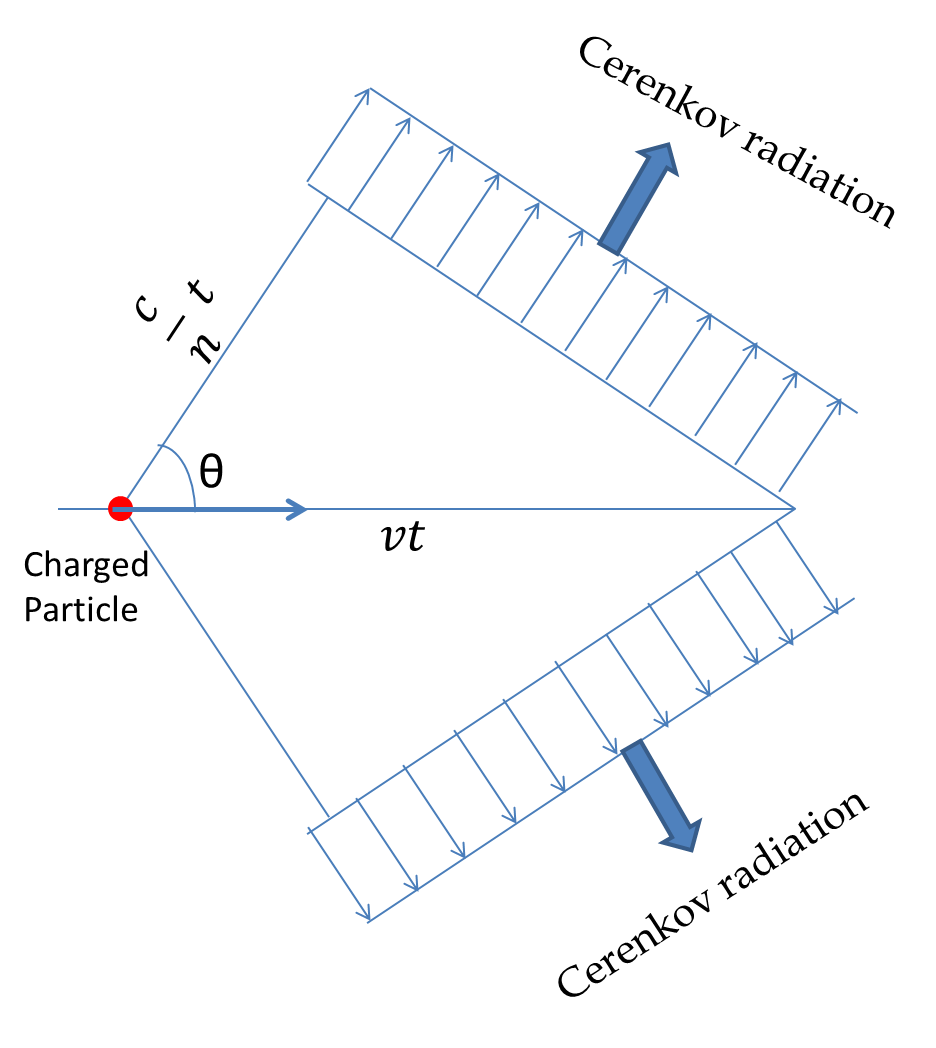Figure 58.5.7. Cerenkov radiation is emitted when a charged particle goes through a dielectric medium in which the speed of the particle exceeds the speed of light in that medium. The measurement of angle $\theta$ can be used to determine the speed of the particle.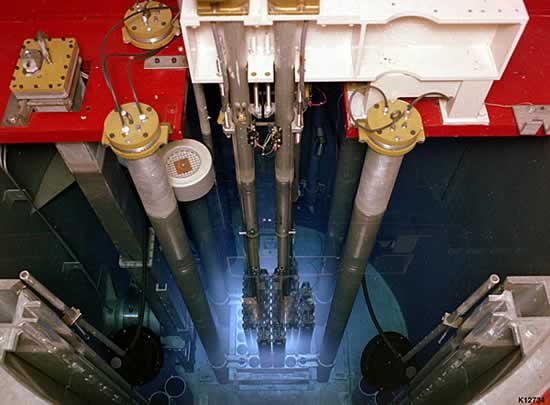Figure 58.5.8. From: http://www.era.anl.gov/advnuclear/nucmeas.html. Description from the webpage: “Neutron Radiography Reactor, Hot Fuels Examination Facility, Idaho National Laboratory. The Neutron Radiography Reactor is a 250 kW TRIGA reactor. It is equipped with two beam tubes and two separate radiography stations and used for neutron radiography irradiation of small test components. The blue glow is caused by Cherenkov radiation. The light water surrounding the core provides ample radiation protection for observers.”

#### Subsubsection58.5.4.1Nuclear emulsion

Nuclear emulsion is a thick transparent medium containing a high concentration of silver halide compound deposited usually on a glass plate. When a particle passes through the medium it reacts with the silver halide and when the plate is developed, you see tracks corresponding to the particle motion. The lengths and curvature of tracks can be measured using a microscope and used to identify particles and their energies.

Photographic plates were extensively used in balloon experiments in the 1930-50s to study cosmic rays. In these experiments photographic plates were sent in balloon into the stratosphere and left there for some time. When the balloons returned, the plates were developed. In one such experiment Cecil Powell and associates found evidence for the particle pion in 1947. The pion had been hypothesized to explain the short range nature of the strong force.

#### Subsubsection58.5.4.2Cloud chamber

A cloud chamber contains vapors of an alcohol or a suitable gas in a supersaturated state. You may either keep the gas in the supersaturated state all the time or bring the gas in the supersaturated state by a sudden expansion of the gas. When a high energy particle passes through the gas it strikes the molecules of the gas and ionizes them. The ionized molecules serve as nucleating sites upon which vapors condense. A track of condensed liquid droplets can be observed in the chamber and photographed. The cloud chamber was invented by Charles T. Wilson in 1912, who used water vapors (Figure 58.5.9) . Now a days, cloud chamber enthusiasts use a chamber filled with isopropyl alcohol vapors cooled by dry ice. Initially, cloud chamber was used to study radioactivity. Throughout 1930s and 1940s cloud chamber played important role in the study of cosmic rays. The cloud chamber was largely replaced by the bubble chamber invented by D. A. Glaser in 1952.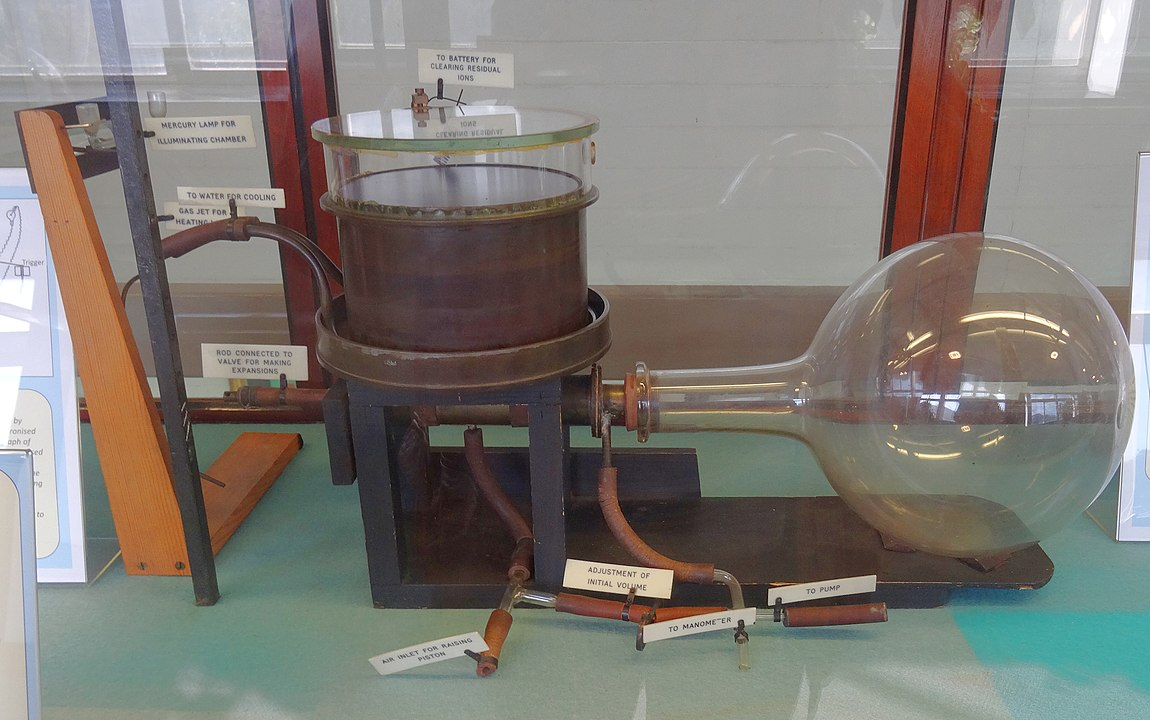Figure 58.5.9. The original C.T.R. Wilson cloud chamber at the Cavendish Lab, Cambridge, England. Credits: Wikimedia Commons.

#### Subsubsection58.5.4.3Bubble chamber

Bubble chamber was invented by D. A. Galser in 1952. The supercooled gas of the cloud chamber is replaced by a superheated liquid, usually liquid hydrogen, in a bubble chamber. In a bubble chamber, usually a cylindrical container is filled with liquid hydrogen and maintained at a temperature slightly below its boiling temperature so that the liquid is not yet boiling. Coincident with the entry of a charged particle a piston in the cylinder is pulled to lower the pressure on the liquid that forces the liquid into a superheated phase. A charged particle through the superheated liquid ionizes molecules of the liquid. The ions form nucleating sites for bubbles which grow and become visible. The track formed by bubbles can be photographed. The analysis of the track of the particle under magnetic field is used to determine various properties of the particle. Bubble chambers have payed important role in particle physics, the most notable success was in the discovery of the neutral current in 1973 at the European facility CERN.

#### Subsubsection58.5.4.4Wire chamber

A drawback of the bubble chamber and other photographed track devices is that the photographs do not lend themselves easily for study by electronic means. A wire chamber is an extension of the proportional counter to many wires between grounded plates as opposed to one wire and one plate as in the Geiger-Muller counter. When a high energy particle passes through the space between plates it ionizes one or more gas molecules. The ionization products, electrons and ions, are attracted to the wire and plate respectively. The signal at the wires give the specific location of the event. In a drift chamber, one make use of the drift time of the ions also to more accurately reconstruct the path. The signals from the wire chambers can be recorded in computers and analyzed using appropriate software. Many more events can be studied in short periods of time than is possible in a bubble chamber. For instance, in a bubble chamber one could study a few events per second, but in wire chambers one can record thousands of events per second.